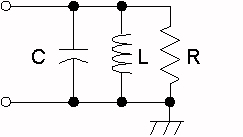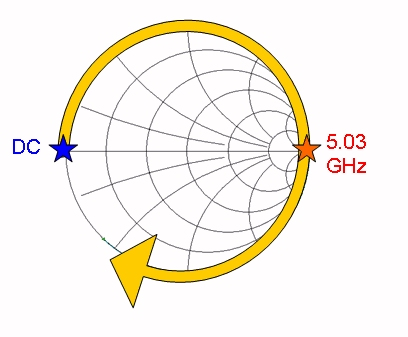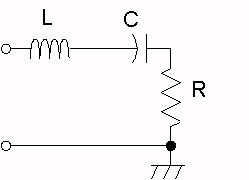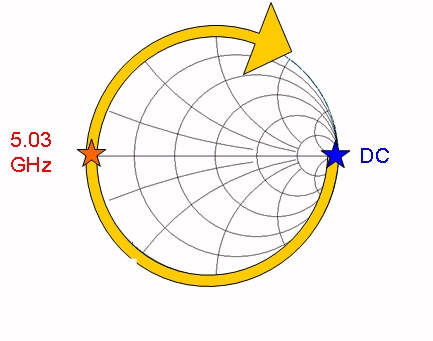# Resonance of RLC Circuits

Resonance is a term used to describe the property whereby a network presents a maximum or minimum impedance at a particular frequency, for example, an open circuit or a short circuit. Resonance is an important concept in microwaves, especially in filter theory. One simple form of resonator is a lumped element RLC circuit, sometime called "tank circuit". Why the term "tank?" Because an LC resonator can store energy in the form of an AC sine wave, much like a pendulum "stores" gravitational energy.

The resonance of a RLC circuit occurs when the inductive and capacitive reactances are equal in magnitude but cancel each other because they are 180 degrees apart in phase. When the circuit is at its resonant frequency, the combined imaginary component of the its admittance is zero, and only the resistive component is observed. The sharpness of the minimum depends on the value of R and is characterized by the "Q" of the circuit.

The formula for resonant frequency (in Excelese) of an LC circuit is:

F=1/(2*PI()*SQRT(L*C/1000))

where F is in GHz, L is in nano-Henries and C is in pico-Farads. Click here to go to our resonant frequency calculator!

Parallel LC resonance
Resonance for a parallel RLC circuit is the frequency at which the impedance is maximum. Plotted below is the special case where the resistance of the circuit is infinity ohms (an open circuit). With values of 1 nH and 1 pF, the resonant frequency is around 5.03 GHz. Here the circuit behave like a perfect open circuit. Note that for R=Z0, at the resonant frequency the response would hit the center of the Smith chart (the arc would still start at the short circuit but would be half the diameter shown). At zero GHz (dc) as well as infinite frequency, the ideal parallel LC presents a short circuit.Parallel Resonance, C=1pF, L=1nH, R=open circuit

Series LC resonance
Resonance for a series RLC circuit is the frequency at which the impedance is minimum. Plotted below is the special case where the resistance of the circuit is zero ohms (an short circuit). With values of 1 nH and 1 pF, the resonant frequency is around 5.03 GHz. Here the circuit behave like a perfect short circuit. Note that for R=Z0, at the resonant frequency the response would hit the center of the Smith chart. At zero GHz (dc) as well as infinite frequency, the ideal series LC presents a open circuit. Thanks for the correction, Rinat!Parallel Resonance, C=1pF, L=1nH, R=short circuit

Author : Unknown Editor